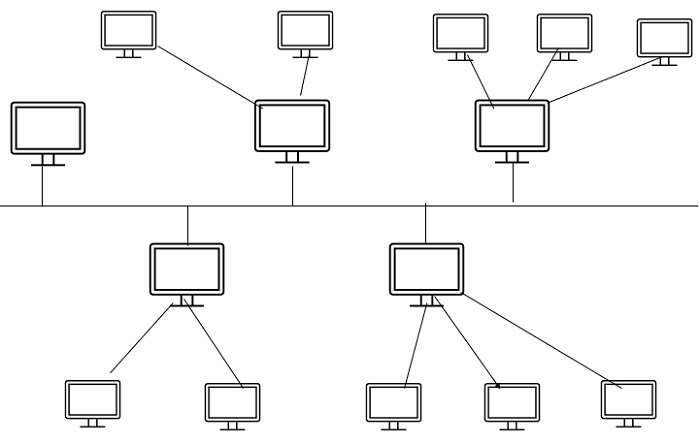# What is Hybrid Topology in Computer Networks?

Computer NetworkInternetMCA

#### Artificial Neural Network and Machine Learning using MATLAB

54 Lectures 4 hours

Topology refers to the arrangement of computer networks. Topology is divided into either physical or logical arrangement. Physical topology refers to the way the computers are connected with each other on a network. Logical topology means the way data is flowing from one computer to another computer.

Now, let see the basic definition of computer network topology, it is the schematic description of network arrangements that are connected through various nodes with the help of lines of connection.

## Types of Network Topology

The different types of network topology are as follows −

• Bus topology
• Ring topology
• STAR topology
• MESH topology
• TREE topology
• Hybrid Topology

Let us understand the concept of Hybrid topology in computer networks.

## Hybrid Topology

It is the combination of two or more different topologies. For example, in a college we have so many departments, let us say one department uses ring topology and another department uses Star topology, connecting these two topologies which results in Hybrid Topology.

Hybrid= Ring+Star## Features

The features of Hybrid Topology are as follows −

• Hybrid Topology is the combination of two or more topologies.

• It inherits the advantages and disadvantages of other topologies also.

The advantages of Hybrid topology are given below −

• It is effective and flexible.

• Troubleshooting is easy.

• Error detecting is reliable.

• It is scalable because the size can be increased easily.